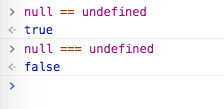#2018 iT 邦幫忙鐵人賽DAY 7
7
Modern Web

## 算術運算子之二

### 正號 `+` 與負號 `-`

``````var a = +10;
var b = -10;

console.log(a);       // 10
console.log(b);       // -10
``````

``````var a = "+10";
var b = "-10";
var c = "Hello";

console.log( +a );      // 10
console.log( -a );      // -10
console.log( +b );      // -10
console.log( -b );      // 10
console.log( +c );      // NaN
console.log( -c );      // NaN
``````

``````+true       // 1
+false      // 0
+null       // 0
+function(val){ return val;}      //NaN
``````

### 遞增 `++` 與遞減 `--`

``````var a = 10;

a++;
console.log(a);       // 11

a--;
console.log(a);       // 10
``````

``````var a = 10;
var b = 10;

a++;
console.log(a);       // 11

++b;
console.log(b);       // 11
``````

``````var a = 10;
var b = 10;

console.log(a++);       // 10
console.log(++b);       // 11

console.log(a);         // 11
console.log(b);         // 11
``````

`++` 放在變數前面時，得到的會是「+1 之後的結果」。

## 比較運算子 (Comparison Operators)

### 「相等」 `==` 與 「全等」 `===`

``````var a = 10;
``````

``````var a = 10;
var b = 100;

console.log( a == b );        // false
console.log( a == 10 );       // true
``````

``````var a = 10;
var b = "10";
``````

``````true == 'true'      // ?

false == 'false'    // ?
``````

``````false == 0    // true
true == 1     // true

[] == []      // false
[] == ![]     // true

[] == ''      // true
[] == 0       // true

[''] == ''    // true
 == 0      // true

 == ''     // false
[''] == 0     // true
````````````null == undefined   // true

[null] == ''        // true
[null] == 0         // true

[undefined] == ''   // true
[undefined] == 0    // true
``````「JavaScript 我真是猜不透你啊～」

``````var a = 10;
var b = "10";

console.log( a == b );      // true

console.log( a === b );     // false
``````

`===` 的情況下，數字的 `10` 與字串的 `"10"`，由於 JavaScript 不會做自動轉型，所以結果會是 `false`，它只會在雙方的數值與型態都相等的狀況下回傳 `true`

### 自動轉型的規則

• 如果其中有一個值為「Boolean」的情況下，會將 `true` 轉型為「數字」的 `1``false` 則會變成數字的 `0`
• 如果遇到字串與數字做比較的情況下，則會將字串透過 `Number()` 嘗試轉型為數字後，再進行比較。
• 如果其中一方是「物件」型別，而另一方是基本型別的情況下，則會先透過物件的 `valueOf()` 方法取得對應的基本型別的值，再進行比較。

• `NaN` 不等於 `NaN`，不管是兩個等號或三個等號都一樣。
• 當兩個物件進行比較時，要看兩者是否指向同一個「實體」，只有在指向同一個「實體」時才會回傳 `true`

### 數值的大於 `>` 與小於 `<`

``````var a = 10;
var b = 11;
var c = 10;

console.log( a > b );       // false
console.log( b > a );       // true

console.log( a > c );       // false
console.log( a >= c );      // true
``````

• 兩者都是數字，則單純就其字面大小比較。
• 如果其中一個是數字，而另一個不是，則會嘗試在背後將另一個先轉為數字後再比較。
• 如果兩者都是字串，則會依照字母的順序 (standard lexicographical ordering) 來進行比較。 [註1]
• 如果其中一者是 boolean 的情況，則會把 `true` 看成 `1``false` 看成 `0` 再進行比較。
• 如果是物件的情況下，則是會先透過物件的 `valueOf()` 方法先求得對應的數值，若物件沒有 `valueOf()` 方法的話，則會透過 `toString()` 轉型再進行比較。

• [註1] standard lexicographical ordering：簡單來說，字串的順序可以想像成「英語字典」中的單字，第一個字母、以 a、b、c ... 到 z 的順序排列；如果第一個字母一樣，那麼比較第二個、第三個乃至後面的字母。需要注意的是，大小寫字母 unicode 裡的順序是不一樣的。

「自動轉型」一直是 JavaScript 初學者很容易搞混的地方，希望透過今天的介紹可以幫助各位理解，如果覺得不過癮的話，還可以參考「JS 真值表### 1 則留言

3

``````console.log( -b );      // -10
``````

Kuro Hsu iT邦新手 4 級 ‧ 2017-12-10 22:42:15 檢舉

`console.log( -b );` 那段確實是我複製時貼錯了，負負得正，所以是 10 才對。